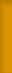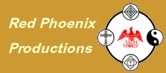HumanHand.com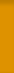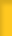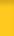:: home:: about us:: ask a question:: handy introduction:: handy how-to guide:: palmistry secrets:: articles & reviews:: ebooks:: certification course:: private readings:: corporate events:: list of palm readers:: handy linksFollow-up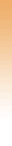After further research, investigation and testing, we finished the Avdeychik/Lagerstrom Fingerprint Coding System - The complete formula and dispensation charts are available here in .PDF Format. » Download File# Fingerprint CombinationsSave This Page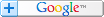## Dispensation of Dermatoglyphic Whorls

by Oleg S. Avdeychik & Kenneth A. Lagerstrom

In modern Dactyloscopy, dermatoglyphic patterns are divided into three basic types:

1. Arch patterns - characterized by the absence of a delta,
2. Loop patterns - characterized by the presence of a delta,
3. Whorl patterns - characterized by the presence of two deltas.
Delta as a concept is a knot, which is made by a junction of three streams of papillary lines. Loop patterns are subdivided into ulnar and radial. Ulnar patterns are those where the feet of the loops are directed towards the little finger. On Radial patterns the feet are directed towards the thumb. Whorls include the "spiral", "bulls-eye", and "double-loop" patterns.

Hands' Nail Phalanx (HNP) prints of a person are set into dactyloscopic formula in the following way:
Numerator - right hand.
Denominator - left hand.
Print arrangement (ordinarily):
- thumb (1), forefinger (2), middle finger (3), fourth finger (4), little finger (5) - for right hand.
- thumb (6), forefinger (7), middle finger (8), fourth finger (9), little finger (10) - for left hand.

Schematically it looks like:
1 | 2 | 3 | 4 | 5 - right hand
----------------------
6 | 7 | 8 | 9 | 10 - left hand

In order to research the dermatoglyphic whorl pattern dispensation at hands' nail phalanges, we used a method where numbers are designated to the fingers as follows:
thumb - 16,
forefinger - 8,
middle finger - 4,
fourth finger - 2,
little finger - 1,

The basic dactyloscopic formula, which consists of a numerator and denominator, was calculated this way: For each whorl pattern on a finger, the finger receives its corresponding number. The numerator contains the sum of all numbers for the right hand +1, and the denominator contains the sum of all numbers for the left hand +1. For example, when there are whorl patterns on all the fingers, the basic dactyloscopic formula will look like this:

16 + 8 + 4 + 2 + 1 +1 = 32
16 + 8 + 4 + 2 + 1 +1 = 32

When there are whorl patterns on the thumb and the fourth finger of right hand and the forefinger and middle finger of the left hand the formula is expressed in the following way:

16 + 0 + 0 + 2 + 0 +1 = 19
0 + 8 + 4 + 0 + 0 +1 = 13

When there are no whorl patterns on fingers the formula is calculated like this:

0 + 0 + 0 + 0 + 0 +1 = 1
0 + 0 + 0 + 0 + 0 +1 = 1

We studied 55,714 HNP prints of both men and women, taken in one region. This database has been formed over 20 years, therefore the results obtained can be considered as stable. Dactyloscopic formulas were calculated according to the method mentioned above and were set into a table (see table 1). In the cells we put the % frequency of a concrete combination's occurrence (number of dactylofiles with a given arrangement of whorl patterns divided by common number of dactylofiles). To exclude occasional occurrences, the frequency of less than 0.05% were designated as 0. Theoretically the maximum number of possible whorl dispensation combinations must be 1,024. As it can be seen from the table 1 in fact there are 233 of them. Many variants count 0.05% or simply do not exist.

For further research the database was divided into 49,743 men and 5,971 women. For each database the HNP whorls' dispensation was obtained in %. In table 2 there is the dispensation of men's database and in table 3, women's. A frequency of less than 0.05% was taken as 0. The number of combinations found is 224 for men and 243 for women. The dispensations were then compared. In table 2 formulas, the frequency of those more than 0.05% is marked as red if the frequency is more for 0.05% than for analogical formulas from table 3, i.e. men's formulas prevail. In the prevalence of women's formulas (table 3), the frequency of occurrences more than 0.05% are marked as blue. This method of coding offered above permits an analysis of whorl dispensation on the fingers of the right hand (horizontally) and those of the left hand (vertically) acc. to formulas. With numbers in the numerator rising, the number of fingers of the right hand with the encoded pattern increases. The same applies to the left hand.

From table 2 it can be seen that the maximum prevalence of men's formulas takes place only at a constant denominator equal to 1. There are 14 such men's formulas when whorl patterns on left hand are absent, and at constant denominators 3-9 there are 9 men's formulas (i.e. when there is a whorl pattern only on the left fourth finger). At constant numerators equal to 23 and 32 (cons. whorl patterns only on the right thumb, middle & fourth finger "23" and all whorl patterns on the fingers of right hand "32") we have maximum number of men's formulas on the fingers of left hand equal to 13.

The same analysis can be made with table 3. What deserves the most attention here is the following result: At constant numerator equal to 1, prevalence of women's formulas is 100% from 15 probable combinations; at constant numerator equal to 11 (whorls on the right forefinger and fourth finger) the number of women's formulas is 14; at constant numerators 3 (whorl on the right fourth finger) and 17 (whorl on the thumb of right hand) the number of women's formulas is equal to 10. Thus we can come to the conclusion that for men the prevalence of whorl patterns on the fingers of the right hand is characteristic (at the given numerator and denominator), and that of women - on the fingers of left hand.

We also conducted research on dispensation of whorl patterns of men & women at the point of absence or presence of radial loops at least on one of the fingers. The resulting % are given in the tables 4-7 where:
Table 4 - dispensation of whorls on men when there are no radial loops on any finger (database 32,318). The red colour is used to mark the formulas with an occurrence frequency for 0.05% that is greater than on table 5.
Table 5 - dispensation of women's whorl patterns when there are no radial loop patterns on any finger (database 4,245). The blue colour is used to mark the formulas with an occurrence frequency for 0.05% that is greater than on table 4.
Table 6 - dispensation of men's whorl patterns when there are radial loop patterns on at least one finger (database 16,539). Red is used to mark the formulas with an occurrence frequency for 0.05% that is greater than on table 7.
Table 7 - dispensation of women's whorl patterns when there are radial loop patterns on at least one finger (database 1,649). Blue is used to mark the formulas with an occurrence frequency for 0.05% that is greater than on table 6.
Occurrence frequencies of less than 0.05% were considered 0. In fact, the number of combinations acc. to table 4 is 211; acc. to table 5 is 256; acc. to table 6 - 184; and acc. to table 7 - 217.

According to table 4 the maximum prevalence of men's formulas takes place at constant denominator of 1 with 15 men's formulas, and at a constant denominator of 3 with 12 men's formulas. At constant numerators 23 & 32 the number of men's formulas is cons. equal to 11 & 17.

From table 5 we show the following result - at a constant denominator equal to 13 & 31 the max. number of women's formulas is 10; at constant numerators 1,2 & 3, the number of women's formulas is 16, 12, & 14. All this confirms the conclusion that for men's formulas the prevalence of whorl patterns is on the right hand (at the above mentioned constants for numerator and denominator), and those of women - on the fingers of left hand. The absence of radial loop patterns has little influence upon the result obtained.

Tables 6 & 7 show the dispensation of whorls on men and women when there are radial loop patterns on the fingers. However because of the small women's database, a comparison is problematic.

A small test study of 200 dactylofiles taken independently and in a seperate region showed almost zero deviation from this data. If this dispensation continues to be analogical to dispensations in other regions and ethnic backgrounds, then it is possible to speak about its global essence.

All the above permits making the following conclusions:

1. HNP whorl patterns can appear only in definite combinations, and submit to their own laws.
2. Whorl patterns prevail on right hands of men and on the left hands of women.
but also raises some questions:
• Why are so few of the theoretical combinations found?
• Can rare dactyloscopic formulas be used to identify genetic anomalies?
The mentioned results served as a background for further research being held at present.

Microsoft Word was used for the following .doc files:
Table 1
Table 2
Table 3
Table 4
Table 5
Table 6
Table 7

Article written: September 24, 1999

Home - About/Contact - Ask a Question - Introduction - Layman's Guide - Handy Secrets - Articles & Reviews - eBooks - Holistic Cheirology™ - Private Readings - Corporate Events - Directory of Professionals - Handy Links - Web Awards - Advertisers - Privacy

Articles: Baby Sign Language - Dispensation of Dermatoglyphic Whorls - Finger and Knuckle Names - Fingernail Problems - The Forer Effect - Free Palm Readings - Hand Transplant Options - Healing Hands - How to Find a Qualified Palm Reader - Making Fingerprints - Palmistry Myths - The Phoenix Logo - PalmTherapy - Simian Line - more....